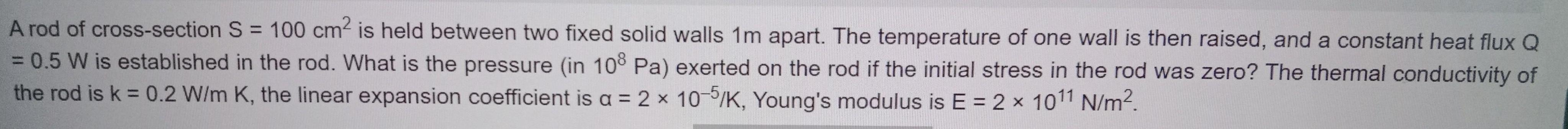Question:

# A rod of cross-section S = 100 cm² is held between two fixed

Last updated: 7/10/2022A rod of cross-section S = 100 cm² is held between two fixed solid walls 1m apart. The temperature of one wall is then raised, and a constant heat flux Q = 0.5 W is established in the rod. What is the pressure (in 10^8 Pa) exerted on the rod if the initial stress in the rod was zero? The thermal conductivity of the rod is k = 0.2 W/m K, the linear expansion coefficient is a = 2 x 10^-5/K, Young's modulus is E = 2 x 10^11 N/m².